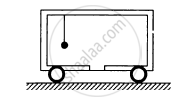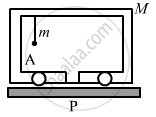Advertisement Remove all ads

# A Car of Mass M is at Rest on a Frictionless Horizontal Surface and a Pendulum Bob of Mass M Hangs from the Roof of the Cart. the String Breaks, the Bob Falls on the Floor, - Physics

Sum

A car of mass M is at rest on a frictionless  horizontal surface and a pendulum bob of mass m hangs from the roof of the cart. The string breaks, the bob falls on the floor, makes serval collisions on the floor and finally lands up in a small slot made in the floor. The horizontal distance between the string and the slot is L. Find the displacement of the cart during this process.Advertisement Remove all ads

#### Solution

The mass of the bob is m.
The mass of the cart is M.

Considering the bob falls at point A.

Initial distance of centre of mass of the system from P is given as
$x = \frac{m \times L + M \times 0}{M + m} = \frac{m}{M + m}L$When the bob falls in the slot, the distance of centre of mass of the system from P becomes zero.

$\therefore \text{ Shift in centre of mass } = 0 - \frac{mL}{M + m}$

$= - \frac{mL}{M + m} \text{ towards left}$

$= \frac{mL}{M + m} \text{ towards right}$

Therefore, the cart moves a distance of
$\frac{mL}{M + m}$ towards right.

Is there an error in this question or solution?
Advertisement Remove all ads

#### APPEARS IN

HC Verma Class 11, 12 Concepts of Physics 1
Chapter 9 Centre of Mass, Linear Momentum, Collision
Q 13 | Page 160
Advertisement Remove all ads
Advertisement Remove all ads
Share
Notifications

View all notifications

Forgot password?